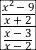## Solving a Rational Equation

This post about solving a rational equation is #15 in a series of posts to help you prepare for the Advanced Algebra and Functions part of the Accuplacer test.

## Question

Solve this rational equation:

Assume x is not equal to -8 or 2/3.

## Solution

What do we mean by rational? The root is ratio. A rational number is a number that can be expressed as quotient — a ratio — of two integers. A rational expression is a quotient — a ratio — of algebraic expressions. A rational equation is an equation comprised of rational expressions.

To get started solving this rational equation, multiply both sides by . That should give you:

Cancel the terms that show up in both numerator and denominator. That’s on the left and on the right. That leaves:

(Yes, I could have just said “cross multiply,” but I hate that expression. It’s a personal quirk.) Now we’re done with denominators.

Distribute:

Subtract  from both sides, and then switch the sides.

Factor:

Set each factor equal to 0:

### Caution

Remember that division by zero yields something undefined, so an x-value that makes a denominator zero is not a valid answer. So you need to make sure that the x values you’ve found do not make one of the denominators in the original question zero. To find those values, set those denominators equal to zero:

Oh, look: Those are the values that the question says are excluded. The question is telling you that a solution that causes the denominator to be zero is not a valid solution. But today that is not your problem, because that’s not one of the answers we found. We found and . Interesting factoid: The official practice question on which this question is based stipulates that x is not equal to -3/2 and then offers -3/2 as an answer choice. If there’s a lesson in that, it may be that it’s a good idea to reread the question before you give your final answer.

To complete the check, substitute  and  into the original equation.

This question is similar to question number 15 in the sample questions for the Accuplacer Advanced Algebra and Functions test.

# How do you simplify a rational expression within a rational expression?

## Question

How do you simplify ?

## Solution

This is a complex fraction, a fraction with fractions in it. To be simplified it needs to have just one numerator and just one denominator.Treat this like the division problem it is. For fractions, division works like this: . . In other words, flip the bottom fraction and multiply. Factor where possible so you can see any places where cancellation is possible. The x – 3 term shows up in both numerator and denominator. Cancel it.

Are we finished? Maybe. That business in the question about being defined? The expression – either the original or the simplified version – is undefined for any value of xthat would make any of the three denominators in the original expression –  ,  , and  — equal to zero. The words “for which it is defined” just mean you can assume you’re not dealing with any of those values of x.

This question is about rational expressions, complex fractions, and domain constraints.